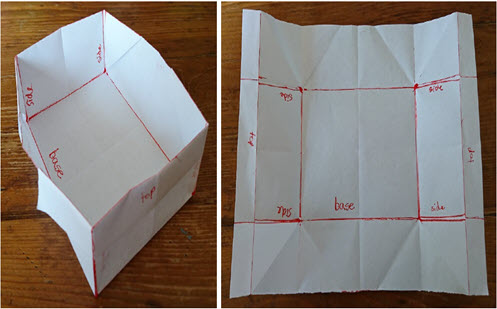Go to website

# reSolve: Quarter Cartons

This lesson challenges students to use algebra and proportional reasoning to investigate how changing the size of a paper square or rectangle impacts the dimensions of a box folded from that paper. Students apply knowledge about nets of 3D objects and explore algebraic relationships through a set of hands-on activities making origami boxes. The context of paper folding encourages students to physically manipulate objects to investigate relationships and demonstrate their findings. The sequence could serve as an introduction to geometric proofs. Each lesson is outlined in detail including curriculum links, vocabulary, materials needed, sample answers, discussion points and student resources. This sequence is part of the reSolve: Mathematics by Inquiry program.

Year level(s) Year 8
Audience Teacher
Purpose Teaching resource
Teaching strategies and pedagogical approaches Mathematics investigation
Keywords factors, area, volume, prisms, nets, origami, dilation, scale, simplification

## Curriculum alignment

Curriculum connections Numeracy
Strand and focus Algebra, Space, Build understanding, Apply understanding
Topics Proportion, rates and ratios, Patterns and algebra, Algebraic expressions, Area, volume and surface area
AC: Mathematics (V9.0) content descriptions
AC9M8M02
Solve problems involving the volume and capacity of right prisms using appropriate units

AC9M8SP02
Establish properties of quadrilaterals using congruent triangles and angle properties, and solve related problems explaining reasoning

AC9M8A01
Create, expand, factorise, rearrange and simplify linear expressions, applying the associative, commutative, identity, distributive and inverse properties

Numeracy progression Understanding units of measurement (P9)
Understanding geometric properties (P6)
Number patterns and algebraic thinking (P7)